# BJT common collector Colpitts oscillator

• Helena Wells
In summary, the conversation is about finding the amplifier gain for a BJT common collector colpitts oscillator. The speaker has searched for hours but has not been able to find the amplifier gain for this specific circuit. They have found it for similar colpitts oscillators with a collector resistor, but the output of this circuit is the collector voltage. They are seeking help and clarification on the difference between the load in the open loop and closed loop configuration and the different types of gain (voltage, current, power). They also mention a video they are watching for more information and offer to provide an LTspice.asc.txt file for further analysis.f
Just a small correction: It is the LOOP GAIN which must be "1"-

Yes, the correct name should be LOOP GAIN.
Closed loop gain and open loop gain are usually used to describe the transfer function of feedback amplifier or feedback control system.By the way, it is sometimes not easy to find the mathematical equation of the oscillator loop gain, so I tried the simplest example below, but I think it is too difficult to derive manually for more complicated situation.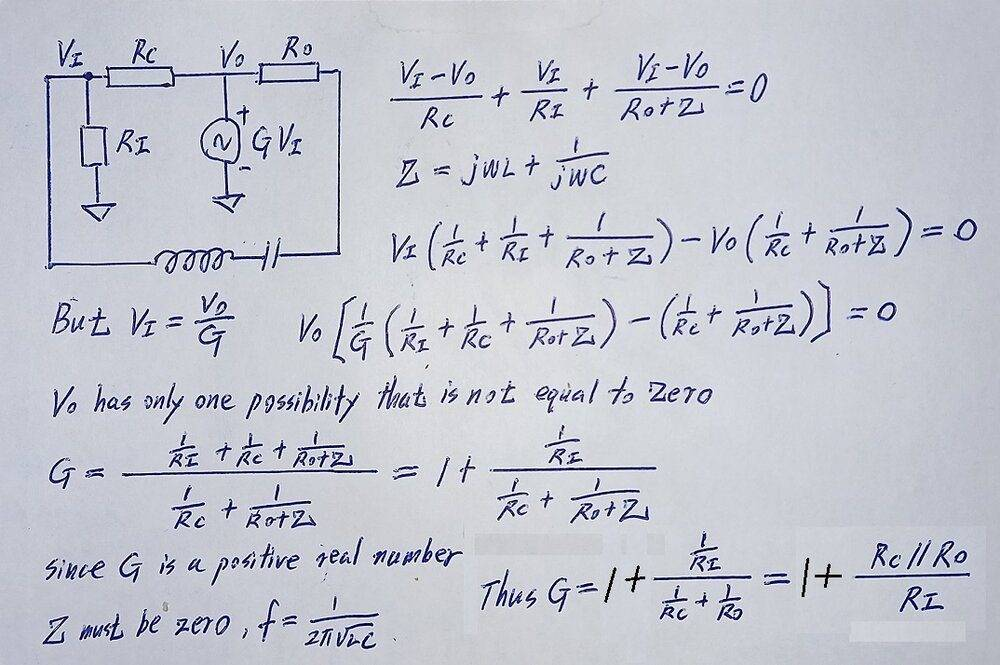Last edited:
The transistor cannot be said to be in Common Collector.
If you view the schematics in the reference provided by the OP, you will see why this implementation is called Common Collector.
http://fourier.eng.hmc.edu/e84/lectures/ch4/node12.html

The component values in the OP circuit are non-optimum in that the amplitude is regulated by crashing hard against the supply rails. That heavy clipping and harmonic distortion is caused by excessive loop gain. The resulting signals are not sinusoidal which makes analysis of the steady state difficult.

By reducing the tank transformer gain, C1=330 pF, C2=100 pF, the circuit can be tamed to the point where the signals are sinusoidal (harmonics more than 40 dB down), the oscillator starts reliably, and the BJT is never turned off completely at any point in the cycle. By using a high resistance base bias (Rth=45k), with a low value of C3 = 10 pF, the signal is attenuated slightly and the influence of transistor parameters on the tank resonator frequency is reduced. Raising the value of the emitter resistor reduces current consumption, and increases the Q of the tank circuit.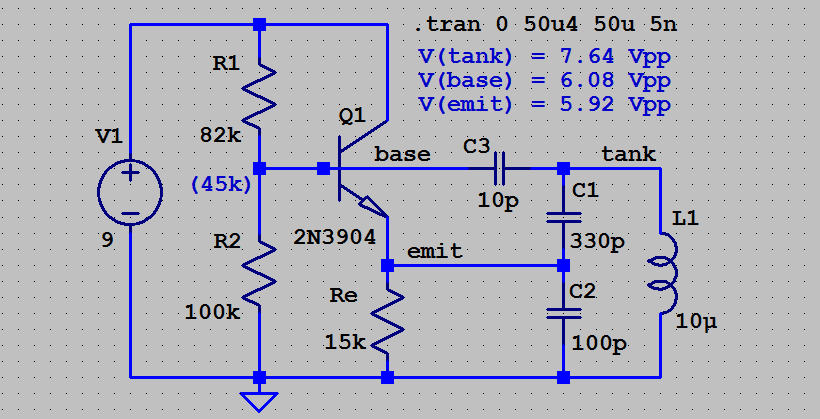There is no inverter in this implementation. The emitter follower is non-inverting, as is the tank circuit transformer. Apart from the power supply, there are only three nodes in the circuit.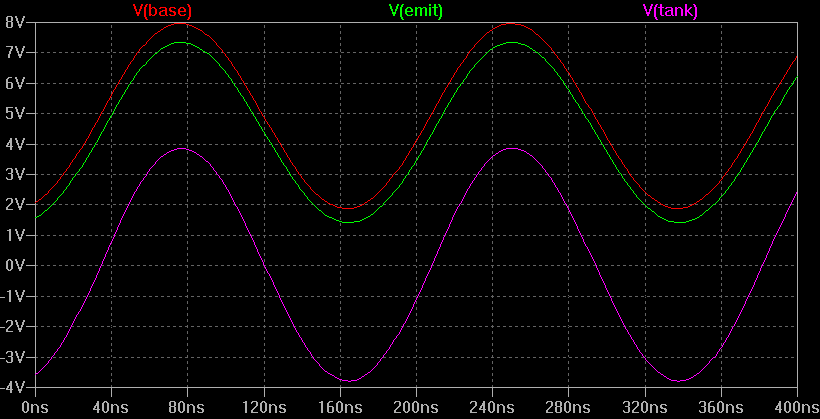The simulation peak-peak amplitude of the sine waves at the three active nodes are as follows. V(tank)=7.64 Vpp; V(base)=6.08 Vpp; V(emit)= 5.92 Vpp. From that a voltage gain audit of the loop shows the gain is distributed as follows.

The tank to base gain; G = 6.08 / 7.64 = +0.796
The C3=10 pF coupling and base bias, Rth=45k, provides base signal current.

The base to emitter gain is; G = 5.92 / 6.08 = +0.974
Which is expected for an emitter follower.

The tank transformer gain is; G = 7.64 / 5.92 = +1.290
As a guide, I would expect; G = (1/C1+1/C2)*C1 = 1.303

The loop gain is the product of those three gain factors. Naturally the three Vpp amplitudes mutually cancel around the loop, to unity.
(6.08 / 7.64) * ( 5.92 / 6.08) * (7.64 / 5.92) = 1.000

•Tom.G
... the amplitude is regulated by crashing hard against the supply rails.
- why do you think it's crashing real hard? The base voltage reaching 5V, the base current increases and discharges the C3 so as to decrease the average emitter current and to stop the oscillation amplitude increase. So the amplitude is just reasonably stabilized, if the transistor is fast even when approaching saturation.

Last edited:
- why do you think it's crashing real hard?
It needs a gain of 1.000; if it had a gain of 2.000 I would expect the BJT to be hard off for half the time. That generates harmonics.
When I simulate the OP circuit (with +5 V supply) I see;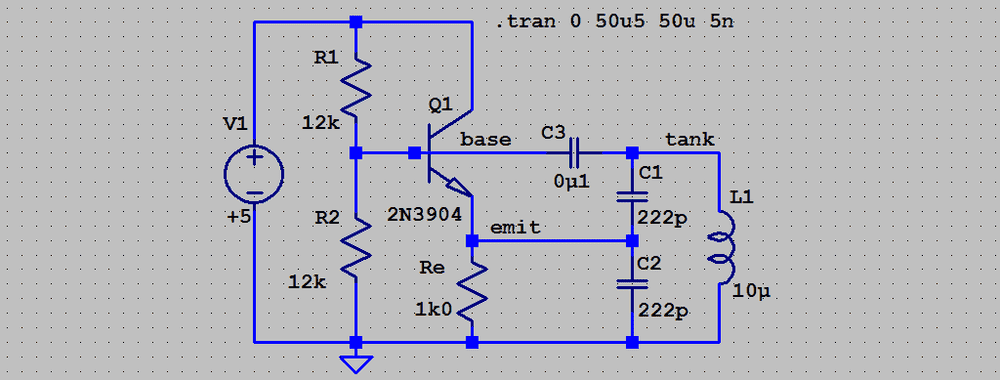Note the 54 mA current spike...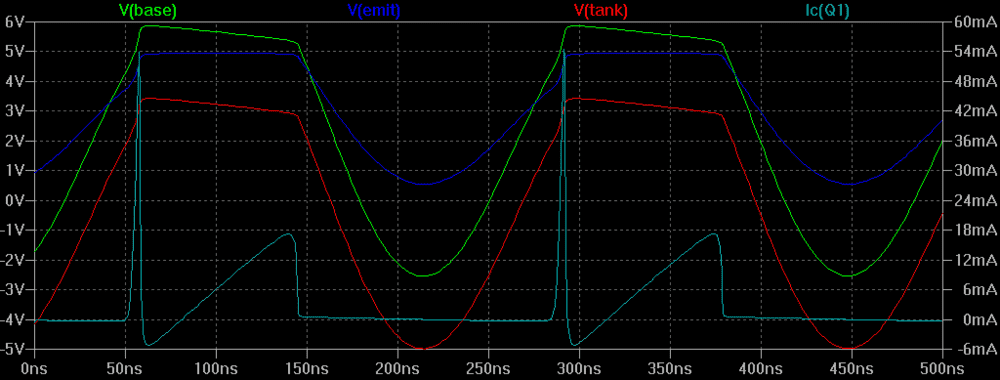Compare that with a tamed version, 1.1 mA, with a +9 V supply.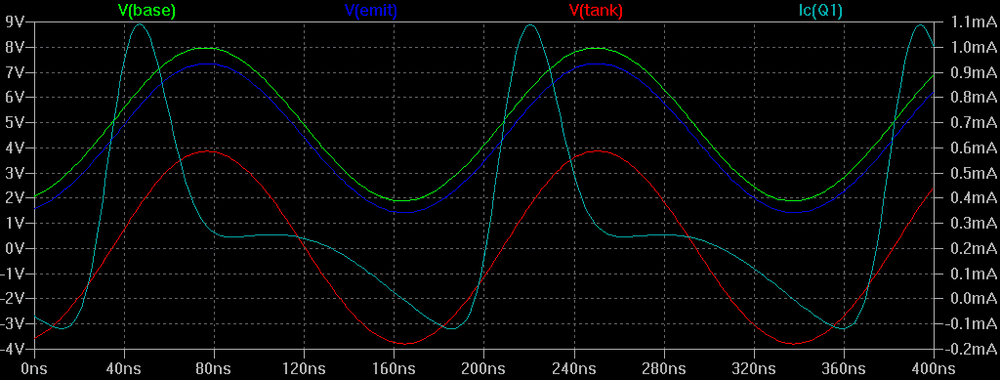•AlexCaledin
- yes, now I see, saturation ought to be avoided.
For amplitude stability, a diode can be added like this: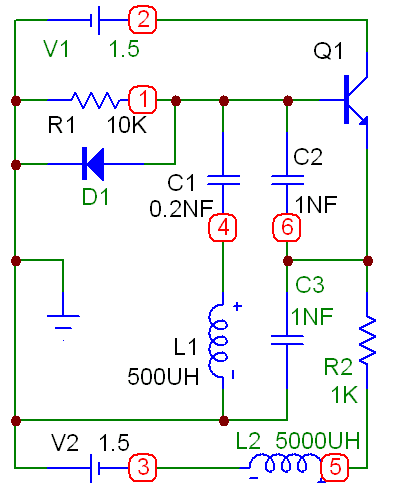- as the oscillation grows, the collector current pulses are suppressed more and more, until the amplitude is stable: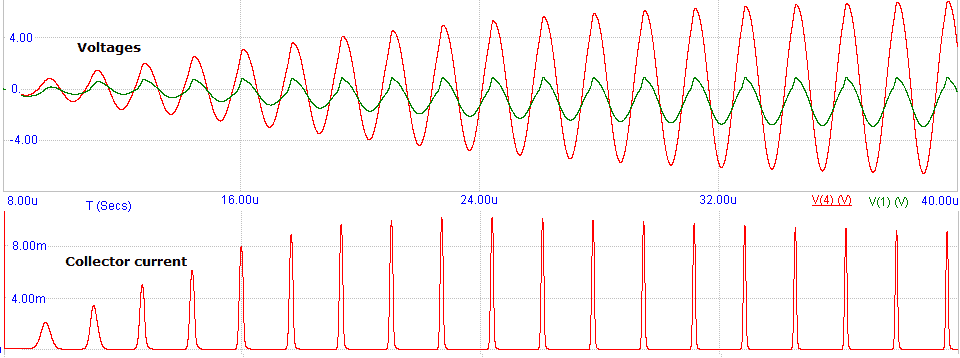(the negative supply is added simply to improve the simulation start, so the capacitors need not to be charged much before the oscillation begins)

Last edited:
- yes, now I see, saturation ought to be avoided.
You do have excessive loop gain, which is evident by the distortion of the sinusoidal tank voltages. Try changing the capacitor ratio, to reduce the gain of the tank, which lowers the harmonic content. That should calm things down a bit.

It seems to me that a negative supply is an expensive way to start a simulation. It should be possible to specify an initial condition, such as 1 mA circulating in the tank, by using the equivalent of a command such as .IC I(L1)=1mA.

Also, the 5mH emitter inductor is very expensive in PCB space and in $. Can you design that out? Maybe increase the emitter resistor value. Looking at the diode, I see it conducts in the same direction as the base. Can the diode be reversed so as to limit on the other half of the cycle, again reducing harmonics? •AlexCaledin and Tom.G You do have excessive loop gain, which is evident by the distortion of the sinusoidal tank voltages. Try changing the capacitor ratio, to reduce the gain of the tank, which lowers the harmonic content. That should calm things down a bit. It seems to me that a negative supply is an expensive way to start a simulation. It should be possible to specify an initial condition, such as 1 mA circulating in the tank, by using the equivalent of a command such as .IC I(L1)=1mA. Also, the 5mH emitter inductor is very expensive in PCB space and in$. Can you design that out? Maybe increase the emitter resistor value.
- that's all quite correct of course! I just tried to show how the diode was moderating the oscillation.
The diode is a sort of peak detector - not just a nonlinear loss resistor.
Of course the final circuit must be like this: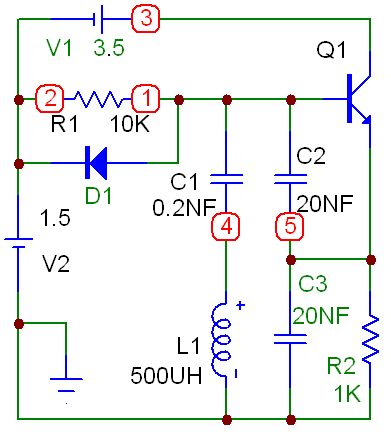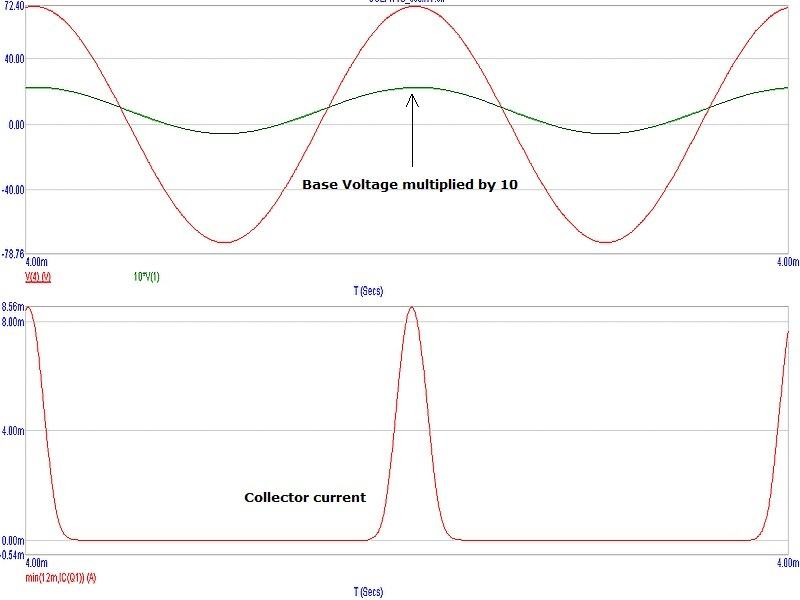- but now it takes more than 1000 cycles to come to something like stationary process.
As for the diode, it's working correct - without it, the transistor current is pretty ugly formed again.
The diode current pulses are about 1mA, way more than the base (20 microAmpere or so).

Last edited:
If an oscillator is to really be a sine wave oscillator, it will need some sort of automatic gain control. Hewlett Packard used a small bulb to regulate gain on their first Wien Bridge audio oscillator. I have also used back-to-back diodes in place of a bulb to give a gentle clamping action in conjunction with a manual gain setting. A useful manual gain control method might be to place a variable resistor in series with the emitter. (Not R2, but in series with emitter alone). This might also help stabilise the gain setting due to the negative feedback created. At the moment the circuit does not have negative feedback.
Another interesting method is to place a variable resistor in parallel with the tuned circuit. Whilst this might be thought to lower the Q and hence destroy the stability, the negative resistance of the circuit cancels the resistance down to some threshold value, when oscillation will gently stop.

•DaveE
This is a typical oscillator - the diode is implementing the automatic gain control exactly the same way.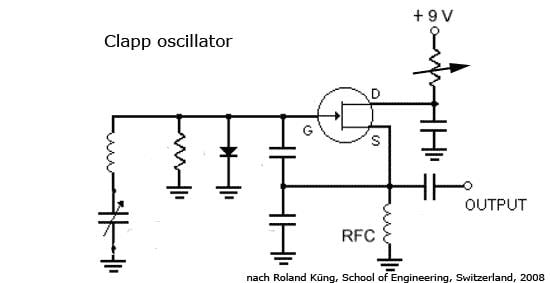Last edited:
•tech99